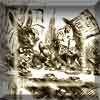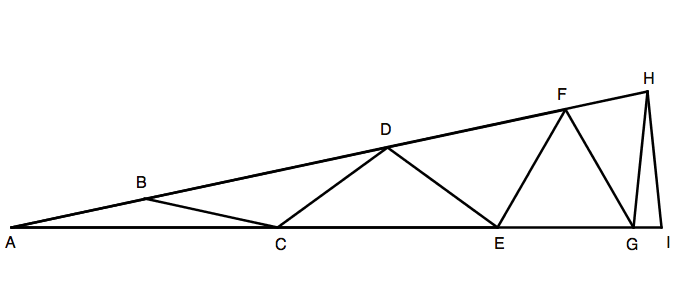#### You may also like### A Problem of Time

Consider a watch face which has identical hands and identical marks for the hours. It is opposite to a mirror. When is the time as read direct and in the mirror exactly the same between 6 and 7?The points P, Q, R and S are the midpoints of the edges of a non-convex quadrilateral.What do you notice about the quadrilateral PQRS and its area?

# Isosceles Seven

##### Age 14 to 16Challenge Level

$AHI$ is an isosceles triangle:Within the triangle are seven other isosceles triangles: $ABC$, $BCD$, $CDE$, $DEF$, $EFG$, $FGH$ and $GHI$.

The eight line segments $AB$, $BC$, $CD$, $DE$, $EF$, $FG$, $GH$, $HI$ are equal in length.

Calculate the three angles of the isosceles triangle $AHI$.

Extension:

Can you construct similar isosceles triangles, made up of a number of smaller isosceles triangles, in which the angles are all whole numbers?

If the isosceles triangle is composed of $n$ isosceles triangles, and angle $BAC = x$, what are the values of the other angles of the triangle?